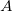# Irrigation Water Costs

Use this form to estimate the how much your irrigation water costs. This can be used to show how much money can be saved by increasing the irrigation efficiency or by using less water per season. Fixed costs from irrigation district assessments, or fixed utility prices that must be paid and do not depend on the quantity of water delivered are not included. Learn more about the units used on this page.

### I Pay by Volume of Water Used (by the gallon)

Common for those on public water utlities. Assumes a flat rate. Some utilities use graded rate scales.
Marginal Water Cost Rate:
Area Irrigated:
Seasonal Water Use (ET):
Irrigation Application Efficiency:
%
Irrigation Water Cost per Season:
\$

### I Just Pay for Pumping Energy Costs

Common for agricultural water users, or those with domestic wells.
Energy Costs per Unit:
Water lift from free water surface to pressure gauge (often the depth of the well while pumping):
Pressure at the gauge and/or after the pump:
Area Irrigated:
Seasonal Water Use (ET):
Irrigation Application Efficiency (based on the irrigation system and operating conditions):
%
Water Pump Efficiency (from the pump chart):
%
Drive Motor Efficiency (Based on the type, size, and condition of the drive motor. Reasonable estimates are below.):
%
Irrigation Water Cost per Season:
\$

## The EquationsAnd:Where:= Area (sq ft for the Utility Equation, acres for the Pumping Energy Equation)= Net Crop Water Use per Season (in)= Water Cost (\$/1000 gallons)= Energy Costs (\$/MJ). Calculated as (\$ per energy unit) / (MJ per energy unit)
Uses:
Electric Motors: 3.6 MJ/kWh, and 92% estimated motor efficiency
Gas Motors: 130.8 MJ/gallon of gasoline, and 30% estimated motor efficiency
Diesel Motors: 146.6 MJ/gallon of diesel, and 45% estimated motor efficiency
Electric Motors: 105.5 MJ/therm (1 therm = 100 cubic ft of natural gas), and 42% estimated motor efficiency= Pressure (psi) + ( Lift (ft) / 2.31 (ft/psi) )= Irrigation Application Efficiency (decimal, or %/100)= Water Pump Efficiency (decimal, or %/100)= Drive Motor Efficiency (decimal, or %/100)

WSU Prosser - IAREC, 24106 N Bunn Rd, Prosser WA 99350-8694, 509-786-2226. Contact Us